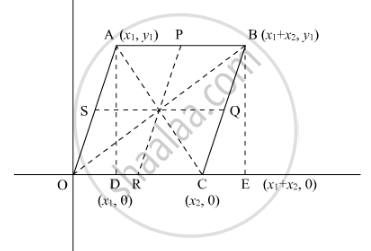Share

# Prove that the Lines Joining the Middle Points of the Opposite Sides of a Quadrilateral and the Join of the Middle Points of Its Diagonals Meet in a Point and Bisect One Another. - CBSE Class 10 - Mathematics

#### Question

Prove that the lines joining the middle points of the opposite sides of a quadrilateral and the join of the middle points of its diagonals meet in a point and bisect one another

#### Solution

Let us consider a Cartesian plane having a parallelogram OABC in which O is the origin.

We have to prove that middle point of the opposite sides of a quadrilateral and the join of the mid-points of its diagonals meet in a point and bisect each other.Let the co-ordinate of A be (x_1,y_1). So the coordinates of other vertices of the quadrilateral are- O (0, 0); B(x_1+x_2,y_1); C(x_2,0)

Let P, Q, R and S be the mid-points of the sides AB, BC, CD, DA respectively.

In general to find the mid-point p(x,y)  of two pointsA(x_1,y_-1)and B(x_2,y_2) we use section formula as,

P(x,y)=((x_1+x_2)/2,(y_1+y_2)/2)

So co-ordinate of point P,

=((x_1+x_2+x_1)/2,(y_1+y_-2)/2)

=((2x_1+x_1)/2,y_1/2)

Similarly co-ordinate of point R,

=(x_2/2,0)

Similary co-ordinate of point S,

=(x_1/2,y_1/2)

Let us find the co-ordinates of mid-point of as,

(((2x_1+x_2)/2+x_2/2)/2,y_1/2)

=((x_1+x_2)/ 2,y_1/2)

Similarly co-ordinates of mid-point of Qs,

=((x_1+x_2)/2,y_1/2)

NOw the mid-pont of diagonal AC,

=((x_1+x_2)/2,y_1/2)

Similarly the mid-point of diagonal OA,

((x_1+x_2)/2,y_1/2)

Hence the mid-points of PR, QS, AC and OA coincide.

Thus, middle point of the opposite sides of a quadrilateral and the join of the mid-points of its diagonals meet in a point and bisect each other.

Is there an error in this question or solution?

#### Video TutorialsVIEW ALL 

Solution Prove that the Lines Joining the Middle Points of the Opposite Sides of a Quadrilateral and the Join of the Middle Points of Its Diagonals Meet in a Point and Bisect One Another. Concept: Area of a Triangle.
S